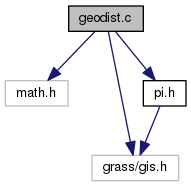GRASS Programmer's Manual  6.5.svn(2014)-r66266
geodist.c File Reference

GIS Library - Geodesic distance routines. More...

`#include <math.h>`
`#include <grass/gis.h>`
`#include "pi.h"`
Include dependency graph for geodist.c:Go to the source code of this file.

## Functions

int G_begin_geodesic_distance (double a, double e2)
Begin geodesic distance. More...

int G_set_geodesic_distance_lat1 (double lat1)
Sets geodesic distance lat1. More...

int G_set_geodesic_distance_lat2 (double lat2)
Sets geodesic distance lat2. More...

double G_geodesic_distance_lon_to_lon (double lon1, double lon2)
Calculates geodesic distance. More...

double G_geodesic_distance (double lon1, double lat1, double lon2, double lat2)
Calculates geodesic distance. More...

## Detailed Description

GIS Library - Geodesic distance routines.

Distance from point to point along a geodesic code from Paul D. Thomas, 1970
"Spheroidal Geodesics, Reference Systems, and Local Geometry"
U.S. Naval Oceanographic Office, p. 162
Engineering Library 526.3 T36s http://stinet.dtic.mil/oai/oai?&verb=getRecord&metadataPrefix=html&identifier=AD0703541

WARNING: this code is preliminary and may be changed, including calling sequences to any of the functions defined here.

(C) 2001-2008 by the GRASS Development Team

This program is free software under the GNU General Public License (>=v2). Read the file COPYING that comes with GRASS for details.

Date
1999-2008

Definition in file geodist.c.

## Function Documentation

 int G_begin_geodesic_distance ( double a, double e2 )

Begin geodesic distance.

Initializes the distance calculations for the ellipsoid with semi-major axis a (in meters) and ellipsoid eccentricity squared e2. It is used only for the latitude-longitude projection.
Note: Must be called once to establish the ellipsoid.

Parameters
 [in] a semi-major axis in meters [in] e2 ellipsoid eccentricity
Returns
always returns 0

Definition at line 52 of file geodist.c.

Referenced by G_begin_distance_calculations().

 double G_geodesic_distance ( double lon1, double lat1, double lon2, double lat2 )

Calculates geodesic distance.

Calculates the geodesic distance from lon1,lat1 to lon2,lat2 in meters.
Note: The calculation of the geodesic distance is fairly costly.

Parameters
 [in] lon1,lat1 longitude,latitude of first point [in] lon2,lat2 longitude,latitude of second point
Returns
distance in meters

Definition at line 212 of file geodist.c.

Referenced by G_distance(), and Vect_line_geodesic_length().

 double G_geodesic_distance_lon_to_lon ( double lon1, double lon2 )

Calculates geodesic distance.

Calculates the geodesic distance from lon1,lat1 to lon2,lat2 in meters where lat1 was the latitude passed to G_set_geodesic_distance_latl() and lat2 was the latitude passed to G_set_geodesic_distance_lat2().

Parameters
 [in] lon1 first longitude [in] lon2 second longitude
Returns
double distance in meters

Definition at line 135 of file geodist.c.

References forms::q, Radians, and y.

Referenced by G_geodesic_distance().

 int G_set_geodesic_distance_lat1 ( double lat1 )

Sets geodesic distance lat1.

Set the first latitude.

Note: Must be called first.

Parameters
 [in] lat1 first latitude
Returns
always returns 0

Definition at line 75 of file geodist.c.

References Radians.

Referenced by G_geodesic_distance().

 int G_set_geodesic_distance_lat2 ( double lat2 )

Sets geodesic distance lat2.

Set the second latitude.

Note: Must be called second.

Parameters
 [in] lat2 second latitidue
Returns
always returns 0

Definition at line 94 of file geodist.c.

References Radians.

Referenced by G_geodesic_distance().